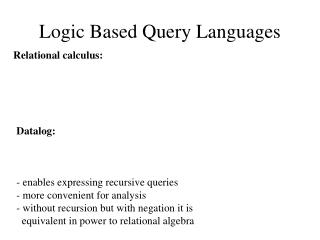DownloadDownload PresentationLogic Based Query Languages

# Logic Based Query Languages

Download Presentation## Logic Based Query Languages

- - - - - - - - - - - - - - - - - - - - - - - - - - - E N D - - - - - - - - - - - - - - - - - - - - - - - - - - -
##### Presentation Transcript

1. Logic Based Query Languages Relational calculus: Datalog: - enables expressing recursive queries - more convenient for analysis - without recursion but with negation it is equivalent in power to relational algebra

2. Datalog Concepts • Atoms • Datalog rules, datalog programs • EDB predicates, IDB predicates • Conjunctive queries • Recursion • Built-in predicates • Negated atoms, stratified programs. • Semantics: least fixpoint.

3. Predicates and Atoms - Relations are represented by predicates - Tuples are represented by atoms. Purchase( “joe”, “bob”, “Nike Town”, “Nike Air”, 2/2/98) - arithmetic, built-in, atoms: X < 100, X+Y+5 > Z/2 - negated atoms: NOT Product(“Brooklyn Bridge”, \$100, “Microsoft”)

4. Datalog Rules and Queries • A pure datalog rule has the following form: • head :- atom1, atom2, …., atom,… • where all the atoms are non-negated and relational. • BritishProduct(X) :- Product(X,Y,P) & Company(P, “UK”, SP) • A datalog program is a set of datalog rules. • A program with a single rule is a conjunctive query. • We distinguish EDB predicates and IDB predicates • EDB’s are stored in the database, appear only in the bodies • IDB’s are intensionally defined, appear in both bodies and heads.

5. The Meaning of Datalog Rules Start with the facts in the EDB and iteratively derive facts for IDBs. Repeat the following until you cannot derive any new facts: Consider every assignment from the variables in the body to the constants in the database. If each of the atoms in the body is made true by the assignment, then add the tuple for the head into the relation of the head.

6. Transitive Closure Suppose we are representing a graph by a relation Edge(X,Y): Edge(a,b), Edge (a,c), Edge(b,d), Edge(c,d), Edge(d,e) b a d e c I want to express the query: Find all nodes reachable from a.

7. Recursion in Datalog Path( X, Y ) :- Edge( X, Y ) Path( X, Y ) :- Path( X, Z ), Path( Z, Y ). Semantics: evaluate the rules until a fixedpoint: Iteration #0: Edge: {(a,b), (a,c), (b,d), (c,d), (d,e)} Path: {} Iteration #1: Path: {(a,b), (a,c), (b,d), (c,d), (d,e)} Iteration #2: Path gets the new tuples: (a,d), (b,e), (c,e) Iteration #3: Path gets the new tuple: (a,e) Iteration #4: Nothing changes -> We stop. Note: number of iterations depends on the data. Cannot be anticipated by only looking at the query!

8. Built in Predicates Rules may include atoms with built-in predicates: ExpensiveProduct(X) :- Product(X,Y,P) & P > \$100 But: we need to restrict the use of built-in atoms in rules. P(X) :- R(X) & X<Y What does this mean? We could use active domain semantics, but that’s problematic. Hence, we require that every variable that appears in a built-in atom also appears in a relational atom.

9. Negated Subgoals Rules may include negated subgoals, but in restricted forms: P(X,Y) :- Between(X,Y,Z) & NOT Direct(X,Z) Bad: P(X, Y) :- R(X) & NOT S(Y) Bad but ok: P(X) :- R(X) & NOT S(X,Y) We’ll rewrite as: S’(X) :- S(X,Y) P(X) :- R(X) & NOT S’(X)

10. Stratified Rules A predicate P depends on a predicate Q if: Q appears negated in a rule defining P. If there is a cycle in the dependency graph, the datalog program is not stratified. Example: p(X) :- r(X) & NOT q(X) q(X) :- r(X) & NOT p(X) Suppose r has the tuple {1}.

11. Subtleties with Stratified Rules Example: p(X) :- r(X) q(X) :- s(X) & NOT p(X). Suppose: R = {1}, and S = {1,2} One solution: P = {1} and Q = {2} Another solution: P={1,2} and Q={}. Perfect model semantics: apply the rules stratum after stratum.